Courses

# Test: Steam Turbines - 3

## 30 Questions MCQ Test Topicwise Question Bank for Mechanical Engineering | Test: Steam Turbines - 3

Description
This mock test of Test: Steam Turbines - 3 for Mechanical Engineering helps you for every Mechanical Engineering entrance exam. This contains 30 Multiple Choice Questions for Mechanical Engineering Test: Steam Turbines - 3 (mcq) to study with solutions a complete question bank. The solved questions answers in this Test: Steam Turbines - 3 quiz give you a good mix of easy questions and tough questions. Mechanical Engineering students definitely take this Test: Steam Turbines - 3 exercise for a better result in the exam. You can find other Test: Steam Turbines - 3 extra questions, long questions & short questions for Mechanical Engineering on EduRev as well by searching above.
QUESTION: 1

Solution:
QUESTION: 2

### What changes occur in pressure and velocity when steam flows through the second row of moving blades of a velocity compounded impulse turbine?

Solution: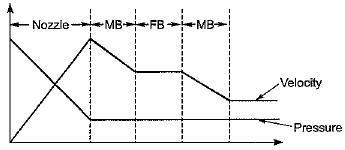QUESTION: 3

### What changes occur in the magnitude of pressure and velocity when steam flows through the nozzles of second stage of pressure compounded impulse turbine?

Solution: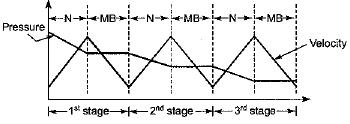QUESTION: 4

The essential merit of a reaction turbine lies in

Solution:
QUESTION: 5

In impulse turbine, the steam expands

Solution:
QUESTION: 6

The expansion of steam, as it flow over the blade of reaction turbine approximated as

Solution:

Expansion in turbine is approximated as isentropic expansion.

QUESTION: 7

In a reaction turbine, a stage is represented by

Solution:

A stage of a reaction turbine consists of each row of blade i.e., one is fixed and other is movable.

QUESTION: 8

A Curtis stage, Rateau stage and a 50% reaction stage in a steam turbine are examples of

Solution:

Curtis stage is a velocity compounded impulse turbine, Rateau stage is pressure compounded impulse turbine.

QUESTION: 9

Compounding of steam turbine is done to

Solution:

Compounding is done to operate the turbine under manageable speed so that turbine can be synchronize with generator. Hence to reduce the speed of turbine compounding is done.

QUESTION: 10

The correct sequence of the given steam turbines in the ascending order of efficiency at their design points is

Solution:
QUESTION: 11

Which one of the following relationship between angles of fixed blades and moving blades corresponds to that of parson’s turbine

Solution:

For Parson’s reaction turbine or 50% reaction turbine
α1 = β2
α2 = β1

QUESTION: 12

In Parson’s reaction turbine the relative velocity at outlet as compared to inlet is

Solution:

Since in the Parson's reaction turbine, the blade of turbine acts as nozzle and hence the relative velocity as exit will be more than that at inlet.

QUESTION: 13

Degree of reaction of an impulse turbine

Solution:

Degree of reaction can be defined as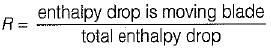Since in impulse turbine all the expansion takes place in nozzle.
Hence enthalpy drop in moving blade = 0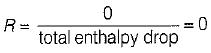QUESTION: 14

In an impulse turbine, the energy supplied to blade per kg of steam is equal to

Solution: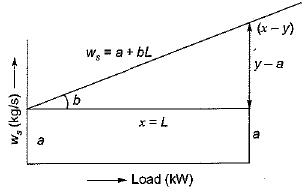Willan’s line shows the steam consumption plotted against the turbine load show a linear relationship with throttle governing
ws = a + bL
where a = no load steam consumption (kg/s)
b = steam rate (kg/kWs)

QUESTION: 15

In Parson’s turbine if α is nozzle angle, then what is the maximum efficiency of the turbine

Solution: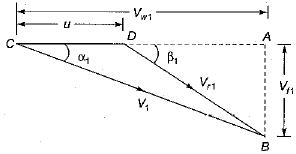For 50% reaction turbine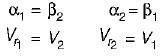Power developed = m(Vw1 + Vw2)U
Input energy =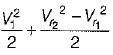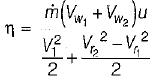Since, Vf2 = V1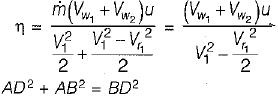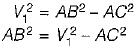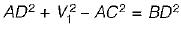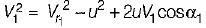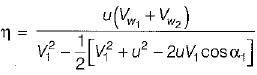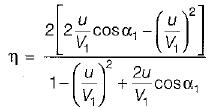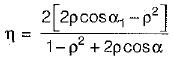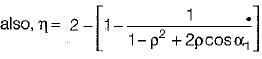For maximum efficiency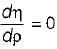By differentiating ρ = u/V1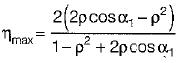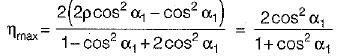QUESTION: 16

Maximum efficiency of a De-Laval turbine is
where α = Nozzle angle

Solution: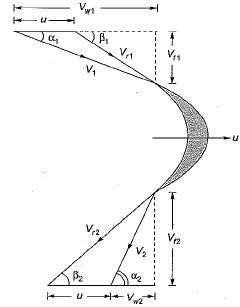Power developed by the Runner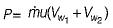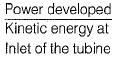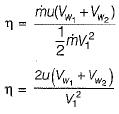For maximum efficiency of impulse turbine.
It is assumed that friction on the turbine blade is neglected i.e.,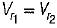and blade is symmetrical i.e., β1 = β2 from the velocity triangle
Vw1 =V1cosα1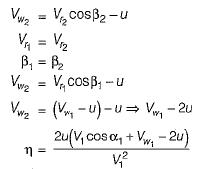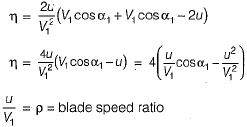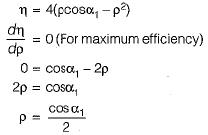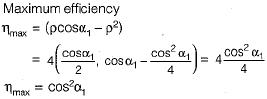QUESTION: 17

Given
V = Absolute velocity of steam entering the blade
α = Nozzle angle

The efficiency of an impulse turbine is maximum when

Solution:
QUESTION: 18

In which one of the following steam turbines, steam is taken from various points along the turbine, solely for feed-water heating?

Solution:
QUESTION: 19

The reheat factor for steam turbines is defined as ratio of

Solution: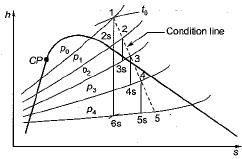Reheat factor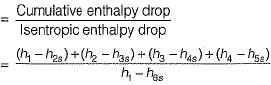QUESTION: 20

What is the value of the reheat factor in multistage turbine?

Solution:

Since constant pressure lines are diverging in forward direction, cumulative enthalpy drop is greater than isentropic enthalpy drop. Hence the reheat factor is greater than unity. Its value lies between 1.04 to 1.08.

QUESTION: 21

Blade erosion in steam turbine takes place

Solution:

If at the exit of turbine, steam is wet i.e., some water particles are suspended in steam, the impact of these water particle leads to blade erosion.

QUESTION: 22

Which of the following method is/are adopted to bring down the speed of an impulse turbine to practical limits?
1. Use of flywheels
2. Use of governor
3. Compounding

Select the correct answer using the code given below:

Solution:

Compounding is done to reduce the speed of impulse turbine to manageable speed.

QUESTION: 23

At which location of a converging-diverging nozzle, does the shock-boundary layer interaction take place

Solution:

At the diverging section of converging diverging nozzle flow velocities are very-very high (M > 1) due to this shock is observed at the diverging portion.

QUESTION: 24

The variation of flow through a convergent- divergent nozzle with variation in exit pressure is represented as

Solution:
QUESTION: 25

The critical pressure ratio for maximum discharge through a nozzle is given by

Solution: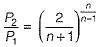P2 = critical pressure at throat
P1 = inlet pressure

QUESTION: 26

The value of critical pressure ratio for superheated steam is

Solution:

Critical pressure ratio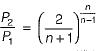For superheated steam
n = 1.3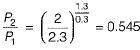QUESTION: 27

The value of critical pressure ratio for initially wet steam is

Solution:

Critical pressure ratio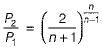For wet steam
n = 1.035 + 0.1x1
Where x1 = initial dryness fraction of steam
Assuming, x = 0.92
n = 1.127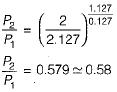QUESTION: 28

The value of critical pressure ratio for initially dry saturated steam is

Solution:

For dry and saturated steam
n = 1.135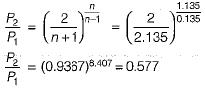QUESTION: 29

For critical pressure ratio, what is the discharge through a nozzle?

Solution:

At critical pressure ratio the discharge becomes maximum and after that the discharge remains constant irrespective of any decrease in pressure ratio.

QUESTION: 30

The presence of air in a condenser

Solution:

Air in the condenser decreases the condensing efficiency of condenser.